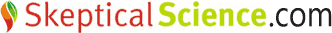# How significance tests are misused in climate science

Guest post by Dr Maarten H. P. Ambaum from the Department of Meteorology, University of Reading, U.K.

Climate science relies heavily on statistics to test hypotheses. For example, we may want to ask whether the global mean temperature has really risen over the past ten years. A standard answer is to calculate a temperature trend from data and then ask whether this temperature trend is “significantly” upward; many scientists would then use a so-called significance test to answer this question. But it turns out that this is precisely the wrong thing to do.

This poor practice appears to be widespread. A new paper in the Journal of Climate reports that three quarters of papers in a randomly selected issue of the same journal used significance tests in this misleading way. It is fair to say, though, that most of the times, significance tests are only one part of the evidence provided.

The post by Alden Griffith on the 11th of August 2010 lucidly points to some of the problems with significance tests. Here we summarize the findings from the Journal of Climate paper, which explores how it is possible that significance tests are so widely misused and misrepresented in the mainstream climate science literature.

Not unsurprisingly, preprints of the paper have enthusiastically been picked up by those on the sceptic side of the climate change debate. We better find out what is really happening here.

Consider a scientist who is interested in measuring some effect and who does an experiment in the lab. Now consider the following thought process that the scientist goes through:

1. My measurement stands out from the noise.
2. So my measurement is not likely to be caused by noise.
3. It is therefore unlikely that what I am seeing is noise.
4. The measurement is therefore positive evidence that there is really something happening.
5. This provides evidence for my theory.
This apparently innocuous train of thought contains a serious logical fallacy, and it appears at a spot where not many people notice it.

To the surprise of most, the logical fallacy occurs between step 2 and step 3. Step 2 says that there is a low probability of finding our specific measurement if our system would just produce noise. Step 3 says that there is a low probability that the system just produces noise. These sound the same but they are entirely different.

This can be compactly described using Bayesian statistics: Bayesian statistics relies heavily on conditional probabilities. We use notations such as p(M|N) to mean the probability that M is true if N is known to be true, that is, the probability of M, given N. Now say that M is the statement “I observe this effect” and N is the statement “My system just produces noise”. Step 2 in our thought experiment says that p(M|N) is low. Step 3 says that p(N|M) is low. As you can see, the conditionals are swapped; these probabilities are not the same. We call this the error of the transposed conditional.

How about a significance test? A significance test in fact returns a value of p(M|N), the so-called p-value. In this context N is called the “null-hypothesis”. It returns the probability of observing an outcome (M: we observe an upward trend in the temperature record) given that the null-hypothesis is true (N: in reality there is no upward trend, there are just natural variations).

The punchline is that we are not at all interested in this probability. We are interested in the probability p(N|M), the probability that the null hypothesis is true (N: there is no upward temperature trend, just natural variability) given that we observe a certain outcome (M: we observe some upward trend in the temperature record).

Climate sceptics want to argue that p(N|M) is high (“Whatever your data show me, I still think there is no real trend; probably this is all just natural variability”), while many climate scientists have tried to argue that p(N|M) is low (“Look at the data: it is very unlikely that this is just natural variability”). Note that low p(N|M) means that the logical opposite of the null-hypothesis (not N: there really is an upward temperature trend) is likely to be true.

Who is right? There are many independent reasons to believe that p(N|M) is low; standard physics for example. However many climate scientists have shot themselves in the foot by publishing low values of p(M|N) (in statistical parlance, low p(M|N) means a “statistically significant result”) and claiming that this is positive evidence that p(N|M) is low. Not so.

We can make some progress though. Bayes' theorem shows how the two probabilities are related. The aforementioned paper shows in detail how this works. It also shows how significance tests can be used; typically to debunk false hypotheses. These aspects may be the subject of a further post.

In the meantime, we need to live with the fact that “statistically significant” results are not necessarily in any relevant sense significant. This doesn't mean that those results are false or irrelevant. It just means that the significance test does not provide a way of quantifying the validity of some hypothesis.

So next time someone shows you a “statistically significant” result, do tell them: “I don't care how low your p-value is. Show me the physics and tell me the size of the effect. Then we can discuss whether your hypothesis makes sense.” Stop quibbling about meaningless statistical smoke and mirrors.

Reference:
M. H. P. Ambaum, 2010: Significance tests in climate science. J. Climate, 23, 5927-5932. doi:10.1175/2010jcli3746.1

Posted by Maarten Ambaum on Friday, 12 November, 2010The Skeptical Science website by Skeptical Science is licensed under a Creative Commons Attribution 3.0 Unported License.